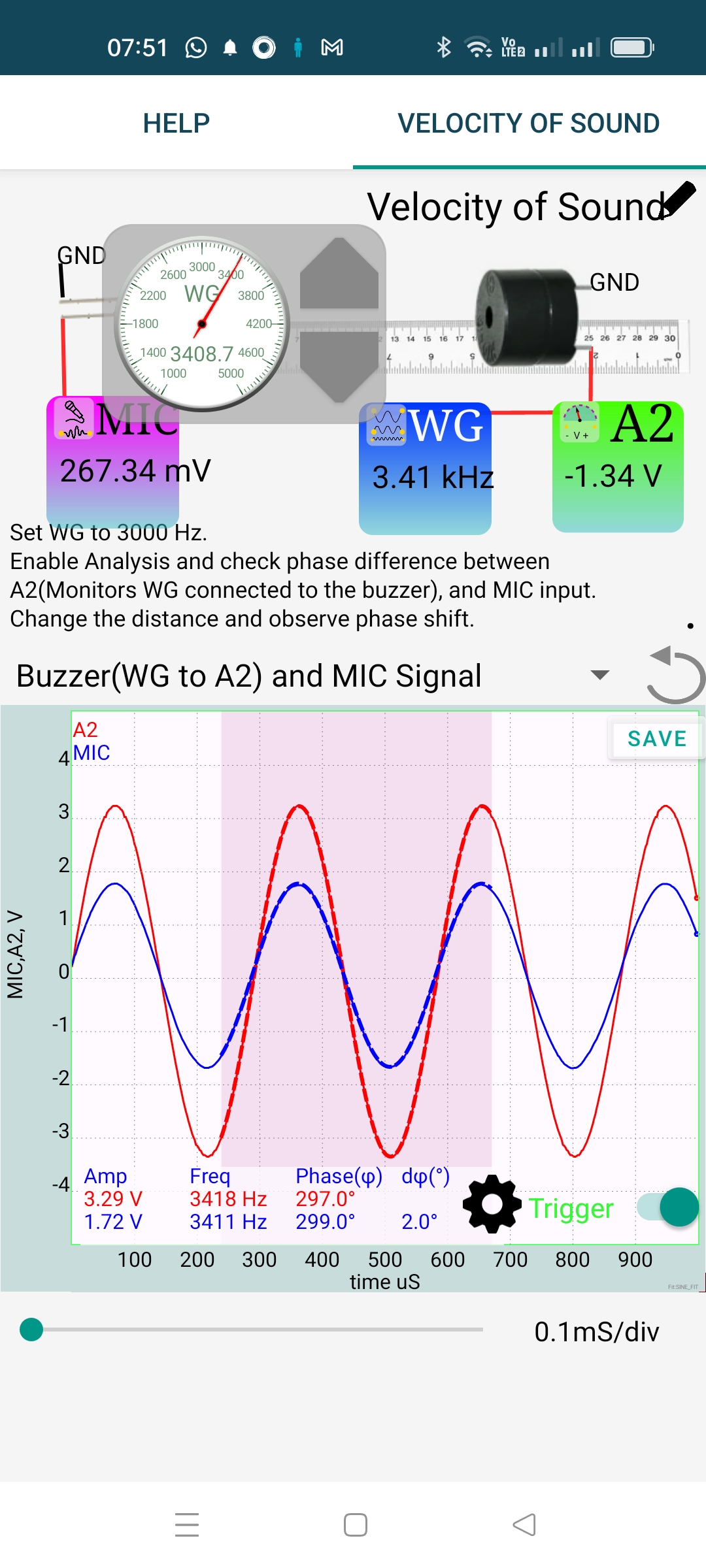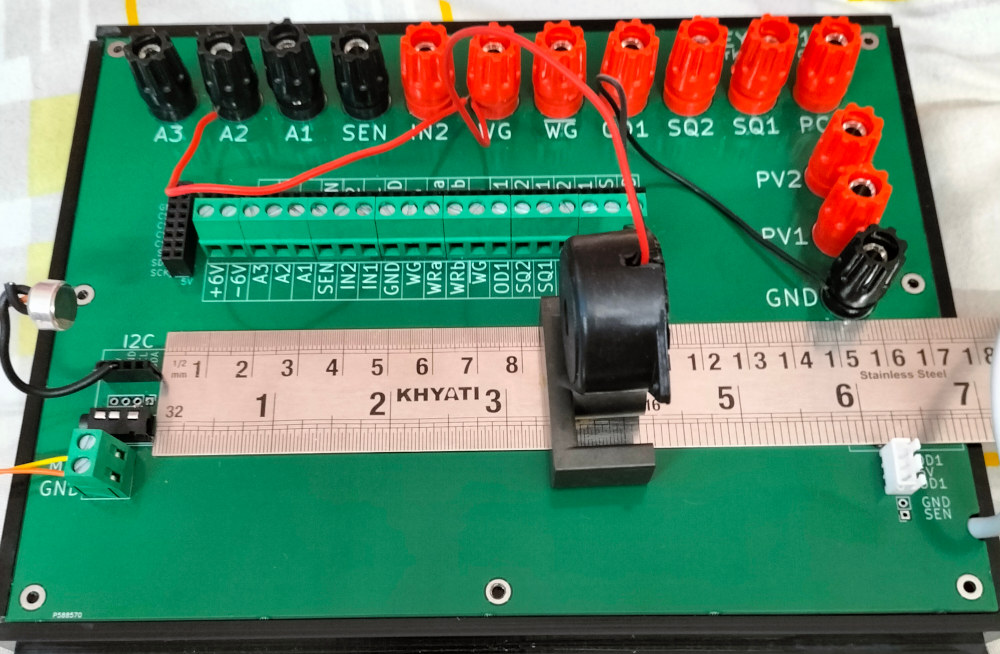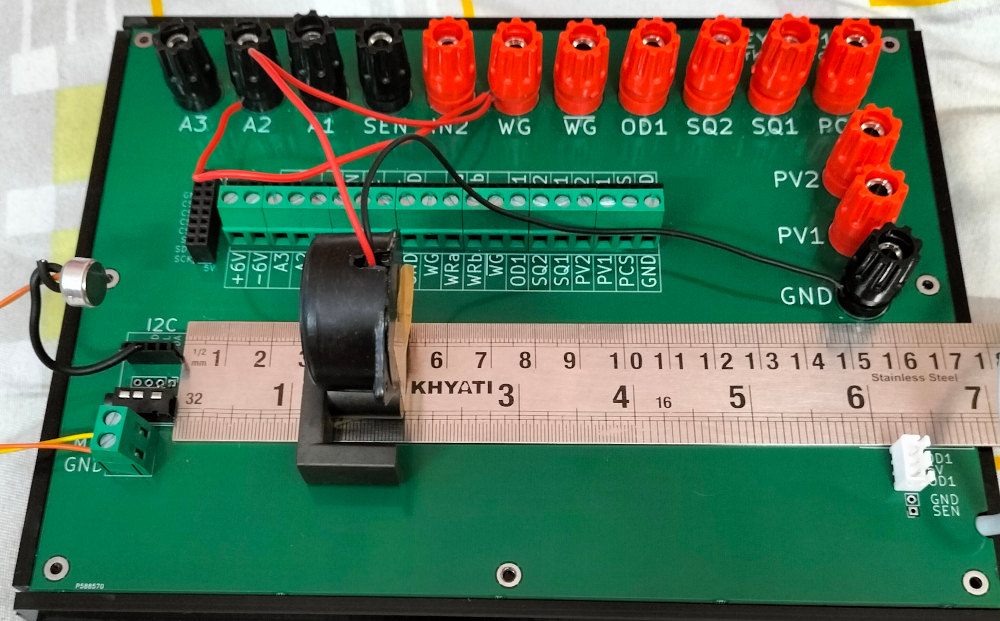# ExpEYES

## Velocity of Sound in Air

Sound waves are longitudinal. It creates compressions and rarefactions in the media along it path. It we place a pressure sensor at some distance from a source of single frequency sound, the sensor output will be a sine wave. The maximum represents the compression point and the minimum the rarefaction point. The output varies with time because the sound wave is travelling.

It we place another sensor along the same line and adjust the distance between the two sensors to make the output waveforms $180^o$ out of phase, the distance between the sensors would be half of the wavelength. Knowing the frequency one can calculate the velocity of sound in air.

It is possible to do this experiment with a single pressure sensor (microphone), if the waveform that generating the sound is available as the reference for phase. The figures below shows the output of WG and MIC, where the distance is adjusted to make both of them in phase. The reading on the scale s around 8.6 cm. The frequency is set to 3400 Hz, somewhere near the resonant frequency of the Piezo buzzer used.

This experiment is performed using the new version of ExpEYES.The distance is adjusted to make the waveforms $180^o$ out of phase as shown below. The scale reading is around 3cm. The difference in distance is 5.4 cm. This gives the velocity = 3400 * 2 * 0.054 = 367 m/s. The results are not very accurate due to the reflections from nearby surfaces.